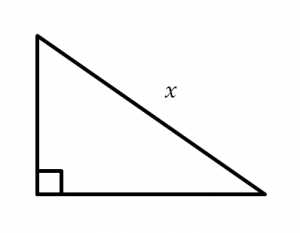Home > Grade 8 > Geometry > Pythagorean Theorem

# Pythagorean Theorem

Directions: What could the lengths of the legs be such that the lengths of the legs are integers and x is an irrational number between 5 and 7?### Hint

What is a wrong answer? How can you use this wrong answer to move towards an answer?

What does it mean to be irrational between 5 and 7?

### Answer

Here are the lengths of the legs
3 and 5,
3 and 6,
4 and 4,
4 and 5,
2 and 5,
2 and 6,
1 and 5,
1 and 6

Source: Daniel Luevanos

## Square Root Expression

Directions: Use the digits 1 to 9, at most one time each, to fill in …